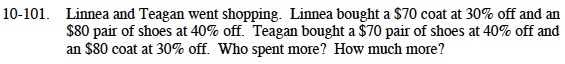### Home > MC1 > Chapter 10 > Lesson 10.2.3 > Problem10-101

10-101.Calculate how much Linnea spent.

If something is 30% off, that means you pay 70%.
10% of $70 is$7
70% = 7(10%)
70% of $70 = 7($7) = $49 Linnea bought her coat for$49.

If something is 40% off, that means you pay 60%.
10% of $80 is$8
60% = 6(10%)
60% of $80 = 6($8) = $48 Linnea bought her shoes for$48.

Linnea spent $48 +$49 = \$90. See if you can use the same method to find out how much money Teagan spent.